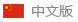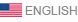Welcome: Henan Ando Magnetics .,LTD
Language:∷# Surface magnetism, remanence and magnetic flux

Is surface magnetism the magnetic force of a magnet? Are magnets with higher surface magnetism better?

Is there any difference and connection between surface magnetism, remanence and magnetic flux?

Why are the values of the same magnet different when measured with a flux meter and a Gauss meter?

Recently, many people have asked these questions. In fact, these questions are related to the surface magnetism, remanence and magnetic flux of permanent magnets. Today we will introduce the similarities, differences and interrelationships of these three concepts.Surface magnetism

Concept: Surface magnetism refers to the magnetic induction intensity of a certain point on the surface of the magnet (then the surface magnetism of the center and the edge is different), which is the value measured by the Gauss meter in contact with a surface of the magnet, not the overall magnetic performance of the magnet.

Measurement: Gaussmeter, also called Teslameter, is generally used to measure the magnetism of magnets. Different manufacturers of Gaussmeters have different Hall sensing elements, and the surface magnetism measured by the same magnet is also different. In addition, it should be noted that the gauss meter measurement standards used in different countries are different.

The surface magnetism is related to the height-diameter ratio of the magnet (the ratio of the height to the diameter of the magnet). The higher the surface magnetism.Magnetic flux

Concept: In a uniform magnetic field with magnetic induction intensity B, there is a plane with area S and perpendicular to the direction of the magnetic field. The product of magnetic induction intensity B and area S is called the magnetic flux passing through this plane, referred to as magnetic flux, symbol "" Φ", the unit is Weber (Wb). Magnetic flux is a physical quantity that represents the distribution of the magnetic field. It is a scalar quantity, but there are positive and negative. Positive and negative only represent its direction. Φ=B·S, when there is an included angle θ between the vertical plane of S and B, Φ=B·S·cosθ

The magnitude of the magnetic flux passing through a plane can be visualized by the number of lines of magnetic field passing through the plane. In the same magnetic field, the higher the magnetic induction intensity, the denser the magnetic field lines. Therefore, the larger the B and the larger the S, the larger the magnetic flux, which means that the number of magnetic field lines passing through this surface is larger. If there are two magnetic fluxes in opposite directions through a plane, the combined magnetic flux at this time is the algebraic sum of the magnetic fluxes in opposite directions.

Measurement: A fluxmeter is an instrument for measuring magnetic flux, and it needs to be matched with a measuring coil (copper wire with a diameter of 0.1-0.5). Helmholtz coil is a device that produces a uniform magnetic field in a small area. Due to the open nature of the Helmholtz coil, other instruments can be easily placed or removed, and visual observation can also be made directly, so it is often used in physical experiments. device. Named after German physicist Hermann von Helmholtz).Remanence

Concept: Remanence refers to the magnetic induction intensity retained in the ferromagnet when the external magnetic field is used to magnetize the ferromagnet to a saturation state and then gradually reduce the external magnetic field to zero, which is called the residual magnetic induction intensity (Br). The remanence is determined by the characteristics of the magnet itself. The remanence of the same magnet is constant under certain conditions and has a single value.

The relationship between remanence and surface magnetism: both are in Gaussian units, but there is no correspondence between surface magnetism and remanence, that is, two magnets with the same remanence may not have the same size of surface magnetism, and the surface magnetism is subject to magnets. shape, size, and magnetization.

1) For two magnets with the same shape, performance and size, the higher surface magnetism has stronger remanence.

2) For two magnets with different shapes, or different performance and size, the size of remanence cannot be determined simply by the level of surface magnetism.

The relationship between remanence and magnetic flux: When the magnetic circuit of the magnet is closed, the flux meter can be used to measure the magnetic flux, and then calculate the remanence. Br=φ/n/s, where: φ represents the magnetic flux, n represents the number of turns of the coil, and s represents the cross-sectional area of the magnet.

No next

## CATEGORIES